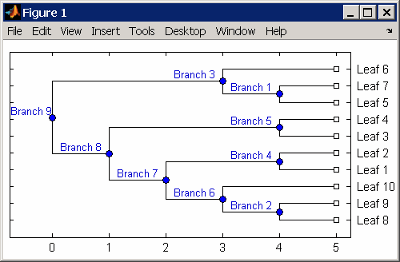# reroot (phytree)

Change root of phylogenetic tree

## Syntax

```Tree2 = reroot(Tree1) Tree2 = reroot(Tree1, Node) Tree2 = reroot(Tree1, Node, Distance) ```

## Arguments

 `Tree1` Phylogenetic tree (`phytree` object) created with the function `phytree`. `Node` Node index returned by the phytree object method `getbyname`. `Distance` Distance from the reference branch.

## Description

`Tree2 = reroot(Tree1)` changes the root of a phylogenetic tree (`Tree1`) using a midpoint method. The midpoint is the location where the mean values of the branch lengths, on either side of the tree, are equalized. The original root is deleted from the tree.

`Tree2 = reroot(Tree1, Node)` changes the root of a phylogenetic tree (`Tree1`) to a branch node using the node index (`Node`). The new root is placed at half the distance between the branch node and its parent.

`Tree2 = reroot(Tree1, Node, Distance)` changes the root of a phylogenetic tree (`Tree1`) to a new root at a given distance (`Distance`) from the reference branch node (`Node`) toward the original root of the tree. Note: The new branch representing the root in the new tree (`Tree2`) is labeled `'Root'`.

## Examples

1. Create an ultrametric tree.

```tr_1 = phytree([5 7;8 9;6 11; 1 2;3 4;10 12;... 14 16; 15 17;13 18]) plot(tr_1,'branchlabels',true)```

A figure with the phylogenetic tree displays.2. Place the root at `'Branch 7'`.

```sel = getbyname(tr_1,'Branch 7'); tr_2 = reroot(tr_1,sel) plot(tr_2,'branchlabels',true)```

A figure of a phylogenetic tree displays with the root moved to the center of branch 7.3. Move the root to a branch that makes the tree as ultrametric as possible.

```tr_3 = reroot(tr_2) plot(tr_3,'branchlabels',true)```

A figure of the new tree displays with the root moved from the center of branch 7 to branch 8.### Topics

Introduced before R2006a

## SupportGet trial now